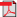RIEB Discussion Paper Series No.2013-27Title

Simple Fixed Point Results for Order-Preserving Self-Maps and Applications to Nonlinear Markov Operators

Abstract

Consider a preordered metric space ( X , d , ⪯ ) . Suppose that d ( x , y ) ≤ d ( x ′ , y ′ ) if x ′ ⪯ x ⪯ y ⪯ y ′ . We say that a self-map T on X is asymptotically contractive if d ( T i x , T i y ) → 0 for all x , y ∈ X . We show that an order-preserving self-map T on X has a globally stable fixed point if and only if T is asymptotically contractive and there exist x , x X such that T i x ⪯ x for all i ∈ ℕ and x T x . We establish this and other fixed point results for more general spaces where d consists of a collection of distance measures. We apply our results to order-preserving nonlinear Markov operators on the space of probability distribution functions on ℝ.

Keywords

Fixed point, Order-preserving self-map, Contraction, Nonlin-ear Markov operator, Global stability

Inquiries

Takashi KAMIHIGASHI